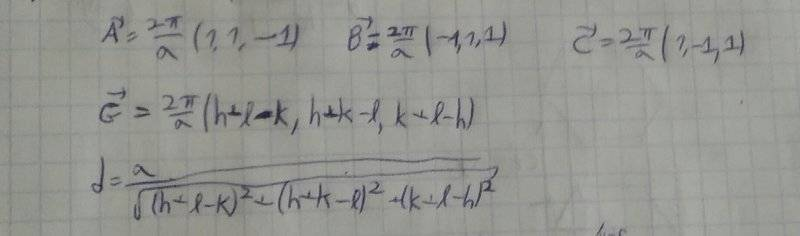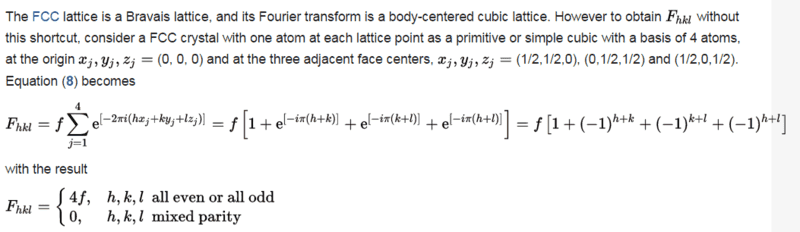# FCC structure factorI tried to treat FCC lattice with a lattice constant a, as a monoatomic lattice.
The reciprocal lattice is a BCC with a lattice constant 2pi/a.
And the structure factor is 1.
But I can treat FCC lattice with a lattice constant a, as a simple cubic lattice,
with 4 atoms in each unit cell. As one can see in Wikipedia:Now the distance between adjacent planes in the reciprocal lattice is
as in a simple cubic: a/sqrt(h^2+k^2+l^2),and I cannot see
how I can get the same wavelengths,according to Bragg's diffraction.

## Answers and Replies

nasu
Gold Member
You d
View attachment 203502 I tried to treat FCC lattice with a lattice constant a, as a monoatomic lattice.
The reciprocal lattice is a BCC with a lattice constant 2pi/a.
And the structure factor is 1.
But I can treat FCC lattice with a lattice constant a, as a simple cubic lattice,
with 4 atoms in each unit cell. As one can see in Wikipedia:
View attachment 203503
Now the distance between adjacent planes in the reciprocal lattice is
as in a simple cubic: a/sqrt(h^2+k^2+l^2),and I cannot see
how I can get the same wavelengths,according to Bragg's diffraction.
You did not calculate any specific values for the lattice spacings if you do you'll see they are the same. Try to calculate the first 5 peaks, for example.